Time: 3 Hours                                                                                                      Max. Marks: 100

NOTE: There are 9 Questions in all.

·      Question 1 is compulsory and carries 20 marks. Answer to Q. 1. must be written in the space provided for it in the answer book supplied and nowhere else.

·      Out of the remaining EIGHT Questions answer any FIVE Questions. Each question carries 16 marks.

·      Any required data not explicitly given, may be suitably assumed and stated.

Q.1       Choose the correct or best alternative in the following:                                         (2x10)

a.       Identify a wavelength most suitable for present day high-speed communication applications.

(A)  650 nm                                        (B)  850 nm

(C)    1330 nm                                      (D)  1550 nm

b.      Free space optical signal propagation is generally not preferred because of

(A)  higher attenuation                          (B)  lower bandwidth

(C)  higher dispersion                           (D)  radiation hazard

c.   What is the typical range of attenuation for a plastic clad silica (PCS) fiber?

(A)    1-10 dB/km                                 (B)  10-50 dB/km

(C)  50-100 dB/km                             (D)  100-200 dB/km

d.   The axial refractive index of the core,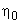of a graded index fiber is 1.50 and the maximum relative refractive index difference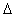is 1%. What is the refractive index of the cladding?

(A)    1.480                                          (B)  1.485

(C)  1.490                                          (D)  1.495

e.   A step-index fiber has a core of refractive index 1.5 and a cladding of refractive index 1.49. The core diameter is 100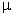m. How many guided modes are supported by the fiber, if the wavelength of light is 0.85m?

(A)     180                                              (B)  570

(C)  1160                                            (D)  2040

f.    Pulse broadening in GI fibers is due to

(A)     Intermodal dispersion                   (B)  Intramodal dispersion

(C)  both (A) and (B)                          (D)  Chromatic dispersion

g.   A fiber-optic link of length 50 km has a rated loss of 0.2 dB/km. The maximum power required to run a photo detector is 20 n w. What power must be supplied by the source?

(A)     20 n w                                         (B) 0.20w

(C)  2w                                           (D) 1w

h.   Two optical fibers with numerical apertures 0.17 and 0.20 are to be spliced. What will be the loss at the joint in the forward direction?

(A)    0 dB                                            (B) 1.41 dB

(C) 1.82 dB                                        (D) 2.50 dB

i.    Which of the following material is not suitable for making an LED?

(A)   GaAs                                             (B) Silicon

(C) InGaAsP                                         (D) GaAlAs

j.    Which of the following detectors give amplified output?

(A)  p-n photodiode                            (B)  p-i-n photodiode

(C)  Avalanche photodiode                  (D)  photovoltaic detector

Answer any FIVE Questions out of EIGHT Questions.

Each question carries 16 marks.

Q.2     a.   Why is parabolic grading preferred for graded index fibers? Explain with the help of  suitable analysis.                                                                    (8)

b.   What do you understand by ‘Direct’ and ‘Indirect’ bandgap semiconductors? Which of the two are more useful for optical source and detector applications and why? Explain.                  (8)

Q.3     a.   With the help of a suitable circuit diagram explain the requirements of an optical source drive circuit for an analog transmission using LASER.        (8)

b.   Explain the principle of working of a non-semiconductor LASER using a suitable diagram.              (8)

Q.4     a.   What is ‘V’ number in connection with optical fibers? What is its significance? Explain.                   (4)

b.   Calculate the number of modes at 820 nm in a graded index fiber having a parabolic index profile, a 25m core radius,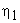=1.48 and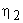=1.46. How does it compare to a step index fiber? (6)

c.   If a step-index fiber has a core of refractive index 1.5, cladding of refractive index 1.48 and a core diameter of 100m, calculate the (i) numerical aperture of the fiber and (ii) pulse broadening per unit length due to multipath dispersion.                                                                                          (6)

Q.5     a.   What do you understand by “splicing”? List and explain the steps involved in splicing of fiber.                                                                     (4)

b.   Compare and contrast the losses caused by Longitudinal, Lateral and Angular displacements in splicing of fibers.                                                  (6)

c.   A 80/125m graded-index (GI) fiber with a NA of 0.25 and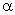of 2.0 is jointed with a 60/125m GI fiber with an NA of 0.21 andof 1.9. The fiber axes are perfectly aligned and there is no air gap. Calculate the insertion loss at the joint for the signal transmission in the forward and backward direction.              (6)

Q.6     a.   What do you understand by “Quantum Efficiency” and responsivity” of an optical detector? Explain.                                                                      (4)

b.   A p-n photodiode has a quantum efficiency of 70% for photons of energy 1.52 x 10-19 J. Calculate (i) the wavelength at which the diode is operating and (ii) the optical power required to achieve a photo current of 3A when the wavelength of incident photons is that calculated in part (i).            (6)

c.   Briefly compare the performance of ‘Si, ‘Ge’ and ‘GaAs’ materials for photo detection application.                                                             (6)

Q.7     a.   Explain the various parameters of an optical link design. How does rise time affect the allowable transmission bandwidth of a given optical system? Discuss.                                                                    (6)

b.   A 15 km optical fiber link uses fiber with loss of 1.0 dB/km. The fiber is joined every kilometre with connectors, which give attenuation of 0.8 dB each. Determine mean optical power, which must be launched into the fiber to maintain mean optical level of 0.3w at the detector.                 (4)

c.   Briefly explain the concept of sub-carrier modulation.                                          (6)

Q.8     a.   What are hetero junctions? How are they useful for superior lasing action? Explain with the help of fundamental principles.                         (8)

b.   Compare and contrast “Homodyne” and “Heterodyne” detection schemes.         (8)

Q.9     a.   With the help of a suitable analysis using ray theory model, determine the number of modes that can propagate in a planar optical wave guide.                                                            (8)

b.   Define the concept of wavelength division multiplexing (WDM). How is it different from FDM and DWDM? Explain.                                               (8)